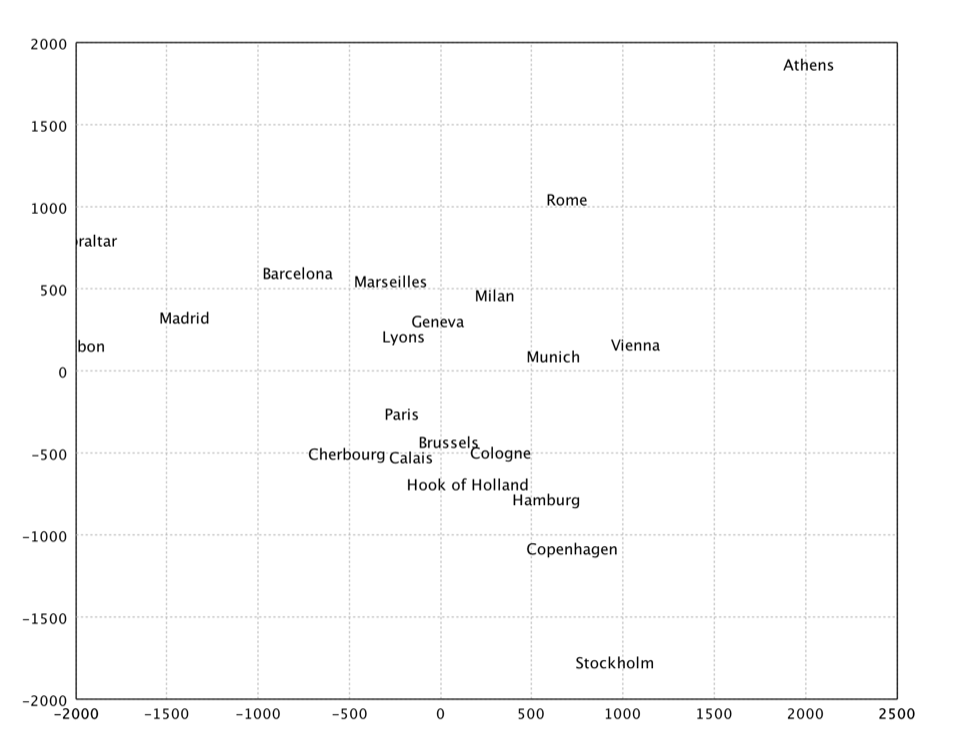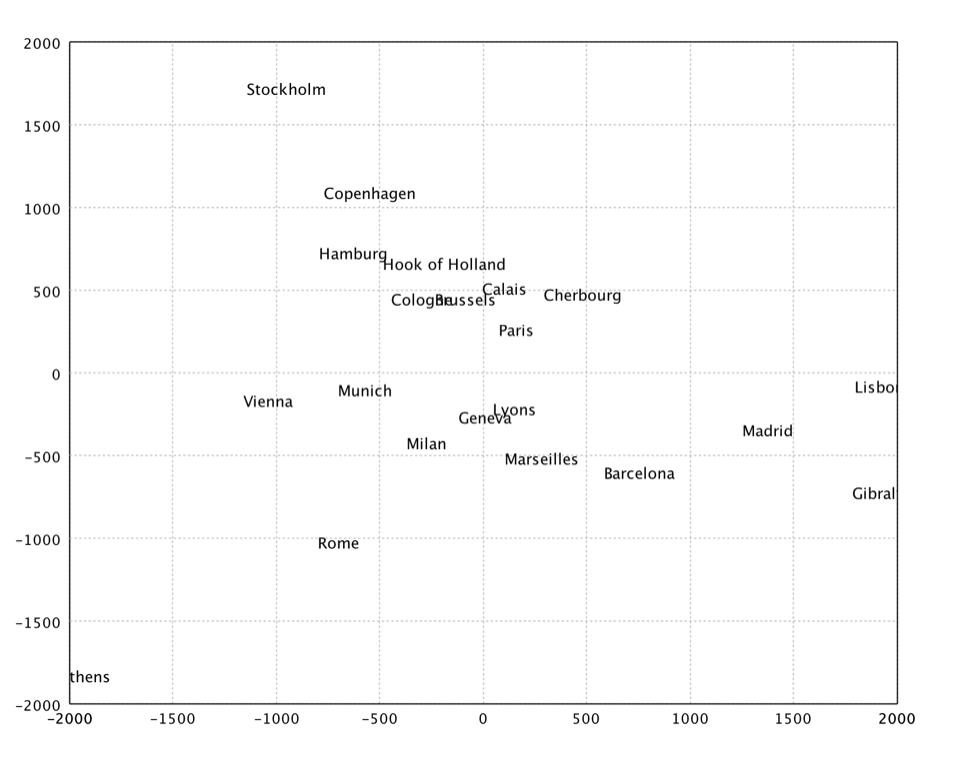Multi-Dimensional Scaling

Multidimensional scaling is a set of related statistical techniques often used in information visualization for exploring similarities or dissimilarities in data. An MDS algorithm starts with a matrix of item-item similarities, then assigns a location to each item in low-dimensional space. For sufficiently low dimension, the resulting locations may be displayed in a graph or 3D visualization.

The major types of MDS algorithms include:

Classical multi-dimensional scaling

It takes an input matrix giving dissimilarities between pairs of items and outputs a coordinate matrix whose configuration minimizes a loss function called strain.

Metric multi-dimensional scaling

A superset of classical MDS that generalizes the optimization procedure to a variety of loss functions and input matrices of known distances with weights and so on. A useful loss function in this context is called stress which is often minimized using a procedure called stress majorization.

Non-metric multi-dimensional scaling

In contrast to metric MDS, non-metric MDS finds both a non-parametric monotonic relationship between the dissimilarities in the item-item matrix and the Euclidean distances between items, and the location of each item in the low-dimensional space. The relationship is typically found using isotonic regression.

Generalized multi-dimensional scaling

An extension of metric multidimensional scaling, in which the target space is an arbitrary smooth non-Euclidean space. In case when the dissimilarities are distances on a surface and the target space is another surface, GMDS allows finding the minimum-distortion embedding of one surface into another.

Classical Multi-dimensional Scaling

Classical multidimensional scaling is also known as principal coordinates analysis. Given a matrix A of dissimilarities (e.g. pairwise distances), MDS finds a set of points in low dimensional space that well-approximates the dissimilarities in A. We are not restricted to using a Euclidean distance metric. However, when Euclidean distances are used, classical MDS is equivalent to PCA.

def mds(proximity: Array[Array[Double]], k: Int, add: Boolean = false): MDS

public class MDS {
public static MDS of(double[][] proximity, int k, boolean positive);
}

fun mds(proximity: Array<DoubleArray>, k: Int, add: Boolean = false): MDS

where proximity is the nonnegative proximity matrix of dissimilarities. The diagonal should be zero and all other elements should be positive and symmetric. For pairwise distances matrix, it should be just the plain distance, not squared. The parameter k is the dimension of output space. If the parameter add is true, the method estimates an appropriate constant to be added to all the dissimilarities, apart from the self-dissimilarities, that makes the learning matrix positive semi-definite. The other formulation of the additive constant problem is as follows. If the proximity is measured in an interval scale, where there is no natural origin, then there is not a sympathy of the dissimilarities to the distances in the Euclidean space used to represent the objects. In this case, we can estimate a constant c such that proximity + c may be taken as ratio data, and also possibly to minimize the dimensionality of the Euclidean space required for representing the objects.

val dist = eurodist.drop(0).toArray
val citys = eurodist.stringVector(0).toArray
val x = mds(dist, 2).coordinates
show(text(citys, x))

var dist = eurodist.drop(0).toArray();
var citys = eurodist.stringVector(0).toArray();
var x = MDS.of(dist, 2).coordinates;
TextPlot.of(citys, x).canvas().window();

val dist = eurodist.drop(0).toArray()
val citys = eurodist.stringVector(0).toArray()
val x = mds(dist, 2).coordinates

In the above example, we apply MDS to a distance matrix of some European cities.MDS

Kruskal's Nonmetric MDS

Kruskal's nonmetric MDS. In non-metric MDS, only the rank order of entries in the proximity matrix (not the actual dissimilarities) is assumed to contain the significant information. Hence, the distances of the final configuration should as far as possible be in the same rank order as the original data. Note that a perfect ordinal re-scaling of the data into distances is usually not possible. The relationship is typically found using isotonic regression.

def isomds(proximity: Array[Array[Double]], k: Int, tol: Double = 0.0001, maxIter: Int = 200): IsotonicMDS

public class IsotonicMDS {
public static IsotonicMDS of(double[][] proximity, int k);
}

fun isomds(proximity: Array<DoubleArray>, k: Int, tol: Double = 0.0001, maxIter: Int = 200): IsotonicMDS

where tol is the tolerance for stopping iterations, and maxIter is the maximum number of iterations.

val x = isomds(dist, 2).coordinates
show(text(citys, x))

var x = IsotonicMDS.of(dist, 2).coordinates;
TextPlot.plot(citys, x).canvas().window();

isomds(dist, 2)Kruskal's Nonmetric MDS

Sammon's Mapping

Sammon's mapping is an iterative technique for making interpoint distances in the low-dimensional projection as close as possible to the interpoint distances in the high-dimensional object. Two points close together in the high-dimensional space should appear close together in the projection, while two points far apart in the high dimensional space should appear far apart in the projection. The Sammon's mapping is a special case of metric least-square multidimensional scaling.

Ideally when we project from a high dimensional space to a low dimensional space the image would be geometrically congruent to the original figure. This is called an isometric projection. Unfortunately it is rarely possible to isometrically project objects down into lower dimensional spaces. Instead of trying to achieve equality between corresponding inter-point distances we can minimize the difference between corresponding inter-point distances. This is one goal of the Sammon's mapping algorithm. A second goal of the Sammon's mapping algorithm is to preserve the topology as best as possible by giving greater emphasize to smaller interpoint distances. The Sammon's mapping algorithm has the advantage that whenever it is possible to isometrically project an object into a lower dimensional space it will be isometrically projected into the lower dimensional space. But whenever an object cannot be projected down isometrically the Sammon's mapping projects it down to reduce the distortion in interpoint distances and to limit the change in the topology of the object.

The projection cannot be solved in a closed form and may be found by an iterative algorithm such as gradient descent suggested by Sammon. Kohonen also provides a heuristic that is simple and works reasonably well.

def sammon(proximity: Array[Array[Double]], k: Int, lambda: Double = 0.2, tol: Double = 0.0001, maxIter: Int = 100): SammonMapping

public class SammonMapping {
public static SammonMapping of(double[][] proximity, int k);
}

fun sammon(proximity: Array<DoubleArray>, k: Int, lambda: Double = 0.2, tol: Double = 0.0001, maxIter: Int = 100): SammonMapping

where lambda is the initial value of the step size constant in diagonal Newton method.

val x = sammon(dist, 2).coordinates
show(plot(citys, x))

var x = SammonMapping.of(dist, 2).coordinates;
TextPlot.of(citys, x).canvas().window();

sammon(dist, 2)Sammon's Mapping